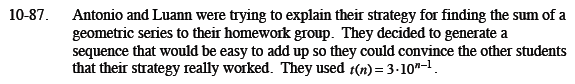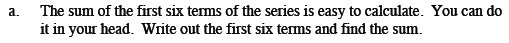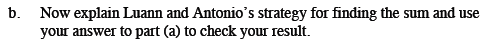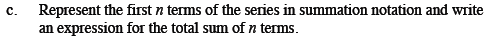Home > CCA2 > Chapter 10 > Lesson 10.2.1 > Problem10-87

10-87.
1. Antonio and Luann were trying to explain their strategy for finding the sum of a geometric series to their homework group. They decided to generate a sequence that would be easy to add up so they could convince the other students that their strategy really worked: t(n) = 3 · 10n−1. Homework Help ✎

1. The sum of the first six terms of the series is easy to calculate. You can do it in your head. Write out the first six terms and find the sum.

2. Now explain Luann and Antonio's strategy for finding the sum and use your answer to part (a) to check your result.

3. Represent the first n terms of the series in summation notation, and write an expression for the total sum of n terms.3 + 30 + 300 + 3,000 + 30,000 + 300,000 = 333,333Write the series 3 + 30 + ... + 300,000 = S(6), twice. Multiply one of them by 10.
Subtract: 10S(6) − S(6) = 9S(6) = 2,999,997. Divide by 9 to get 333,333.$\sum_{k=1}^{n}{(3\cdot10^{k-1})}$